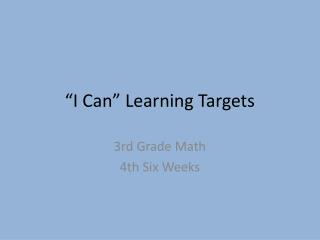DownloadDownload Presentation“I Can” Learning Targets

# “I Can” Learning Targets

Télécharger la présentation## “I Can” Learning Targets

- - - - - - - - - - - - - - - - - - - - - - - - - - - E N D - - - - - - - - - - - - - - - - - - - - - - - - - - -
##### Presentation Transcript

1. “I Can” Learning Targets 3rd Grade Math 4th Six Weeks

2. TNCore Focus Standards 2012-13 • Represent and solve problems involving multiplication and division. • Understand properties of multiplication and the relationship between multiplication and division. Dropped from 2012-13 TCAP: SPI 0306.1.1, SPI 0306,1.3, SPI 0306.1.6, SPI 0306. 1.8, SPI 0306.3.4, SPI 0306.4.2, SPI 0306.4.3, SPI 0306. 5.3

3. 4th Six Weeks Pacing Guide Lessons: • 12.1-12.2 • TN-7 • 12.3-12.4 • TN-8 • 12.5-12.10 • 13.1-13.2 • 13.5 • 14.1-14.3 • TN-9 • 14.4 • TN-10 • 14.5-*14.6 *Note: Dropped from 2012-13 TCAP: SPI 0306.1.6 (Lesson 10.2, 10.6, 10.7, 10.8, 14.6 and TN Handbook: pg. TN31) TNCore Focus Standards: #1 Represent and solve problems involving multiplication and division. #2 Understand properties of multiplication and the relationship between multiplication and division.

4. “I Can”Lesson 12.1-12.10 • I can describe the meaning of … • Denominator (like and unlike) • Numerator • Unit Fraction • I can divide shapes into equal parts to make fractions. • I can name parts of a fraction. • I can name equal fractions. • I can use symbols to show more, less, or equal while using fractions. • I can use a number line to order fractions. • I can compare fractions using drawings, models, and benchmark fractions. • I can identify fractions as part of a whole or a set, a point on a number line, and as division of two whole numbers. • I can add fractions with like denominators. • I can subtract fractions with like denominators. • I can solve a problem that has missing or extra information by using patterns. Note: Use TN-7 after lesson 12.2; use TN-8 after lesson 12.4. (see next slide)

5. “I Can”TN-7; TN-8 • I understand that ½, 1/3, and ¼ represent numbers called unit fractions. • I understand that when a whole is divided into equal parts, the sum of all the parts add up to one. • I can name and identify fractions that are less than, equal to, or greater than one.

6. “I Can”13.1-13.2; 13.5 • I can describe the meaning of “decimal”. • I can write a fraction and a decimal using a picture. • I can match fractions of equal value when I hear them spoken, written, modeled, or drawn in a picture/illustration. • I can count dollars and coins up to five dollars. • I can solve a problem that has missing or extra information by using patterns. Note: Teach 13.3 and 13.4 after TCAP (see Tennessee Teacher’s Program Overview, pg. T141)

7. “I Can”Lesson 14.1-14.6 • I can describe the meaning of… • *capacity • *gram • *liquid measures • *mile • *ounce • *area • I understand that all measurements require units. • I can use a ruler to measure to the nearest inch and ½ inch. • I can choose the correct tool to measure. • I can estimate measurements. • I can choose the correct unit of measurement using inches, feet, yards, and miles. • I can choose the correct unit of capacity using pint, quart, gallon, and cup. • I can choose the correct unit of weight using ounce, pound, and ton. • I can identify and use vocabulary to describe two-and three-dimensional shapes. *Note: Dropped from 2012-13 TCAP: SPI 0306.1.6 (Lesson 10.2, 10.6, 10.7, 10.8, 14.6 and TN Handbook: pg. TN31) Note: Use TN-9 after lesson 14.3; use TN-10 after lesson 14.4. (see next slide)

8. “I Can”TN-9; TN-10 • I can measure and write measurements that use mixed units (like feet and inches; or feet and yards; or meters and centimeters) • I can recognize the use of fractions in liquid measures.